# Polar space

Letbe a set of points with a non-empty collection of distinguished subsets of cardinality, called lines. Such a structure is called a polar space if for each lineofand each point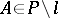the pointis collinear either with precisely one or with all points of. A non-degenerate polar space is one which has no points that are collinear with other points (i.e. it is not a "cone" ). A polar space is linear if two distinct lines have at most one common point.

Examples arise by taking a projective space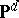(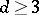to get something non-trivial) with a polarity defined by a non-degenerate bilinear form. Take the subsetof absolute points (also called isotropic points), i.e.. The lines inare the projective lines ofwhich are entirely in. The name "polar space" derives from this class of examples.

A subspace of a polar space is a subset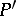ofsuch that ifandandare collinear and unequal, then the whole line throughandis in. A singular subspace of a polar space is one in which every pair of points of it is collinear.

A Tits polar space of rank,, is a set of pointstogether with a family of subsets, called subspaces, such that:

i) a subspace together with the subspaces contained in it is a-dimensional projective space;

ii) the intersection of two subspaces is a subspace;

iii) given a subspaceof dimension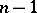and a point, there is a unique subspacecontainingsuch thathas dimension; the spacecontains all points ofthat are joined toby a line (a subspace of dimension 1);

iv) there exist at least two disjoint subspaces of dimension.

The Tits polar spaces of rank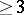are known , [a2] and are classical, i.e. they are Tits polar spaces arising from a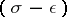-Hermitian form (cf. Sesquilinear form) or a pseudo-quadratic form on a vector space over a division ring, by taking as subspaces the totally-isotropic subspaces of the form (of Witt index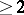). In particular, the subspaces of a finite polar space of rankare the totally-isotropic subspaces with respect to a polarity of a finite projective space or the projective spaces in a non-singular quadric in a finite projective space.

Every non-degenerate polar space is linear, and if for a non-degenerate polar space of finite rank all lines have cardinality, then the singular subspaces define a classical polar space [a3].

A non-degenerate polar space is either classical or a generalized quadrangle (cf. Quadrangle, complete).